# Interpreting Multiplication

 Listen to this Lesson:

In units on multiplying and dividing fractions, fifth-graders begin interpreting multiplication as scaling, by comparing the size of a product to the size of one factor, given the size of the other factor, without calculation.

Lessons on fractions can be challenging for students, and interpreting multiplication as scaling with fractions is no exception to this. To help make the learning process easier and more fun at the same time, math teachers can use a variety of teaching strategies and activities.

In this article, we bring you a few awesome strategies and activities to teach this topic. Use them in your class and you’ll be guaranteed to get students excited about interpreting multiplication!## Strategies to Teach Interpreting Multiplication

### Review Equivalent Fractions

You can start your lesson on interpreting multiplication by reviewing a few prior concepts, such as equivalent fractions. Remind students that equivalent fractions are fractions that have the same value. They may look different from each other, but they name the same fraction.

Equivalent fractions are the result of multiplying or dividing the numerator and the denominator by exactly the same non-zero whole number. When changed to their decimal form, these equivalent fractions will also result in the same number. Present a few examples, such as: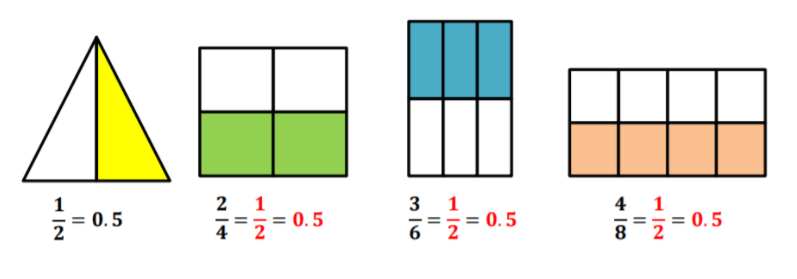#### Comparing the First Factor and the Product

Write a few fractions on the whiteboard that represent equivalent fractions, ex: 1⁄2, 2⁄4, 3⁄6 and 4⁄8. Ask students to think of the way we obtained these fractions. Based on what was explained on using multiplication and division to find equivalent fractions, we can infer the following:This means that 1⁄1, 2⁄2, 3⁄3 and 4⁄4 are all equal to 1. Therefore, multiplying the numerator and denominator of 1⁄2 with the same non-zero whole number will result in the same number.

In other words, we can find equivalent fractions by multiplying the same number by its numerator and denominator. This is because when we multiply the numerator and denominator by the same number, the value of the fraction doesn’t change.

Add that we can also find equivalent fractions of a given fraction by dividing both the numerator and the denominator by the same number. We usually do this when we want to reduce the given fraction to a smaller equivalent fraction.

Any number divided by itself is always equal to one. Any number multiplied by one is the number itself. You can provide a few more examples for students to practice.

### Expressing Fractions as Equivalent Decimals

Point out that one very important skill that students need to master is how to change fractions to decimals. Add that we will use the concept of equivalent fractions when expressing fractions as equivalent decimals.

Most students probably know that 1⁄2 equals 0.5 or that 2⁄10equals 0.2. But what about other fractions? For instance, what is the equivalent decimal of 1⁄5? Provide a worked example to show students how to express fractions as equivalent decimals.

Point out that we can use the following steps in this process: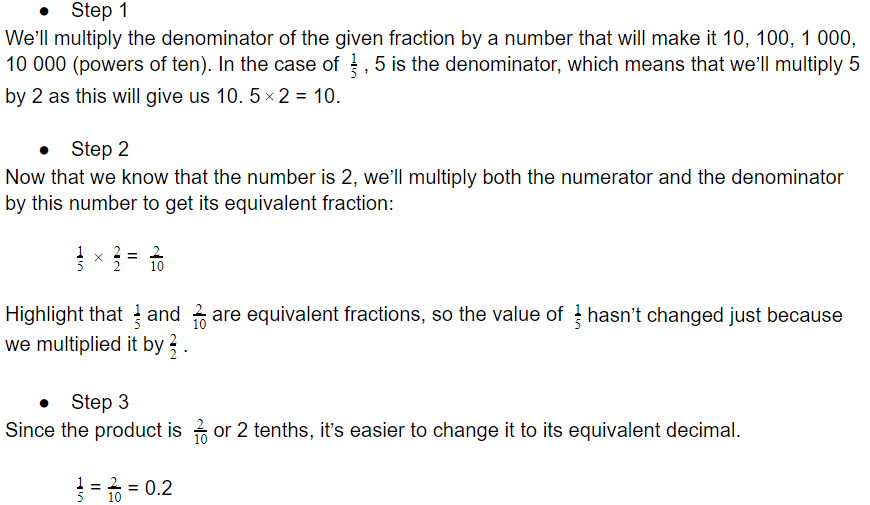### Multiplication as Scaling with Fractions

Write a few multiplication problems on the whiteboard involving fractions, where one factor is present in all of the problems. Ask students to observe the multiplication problems and to try to guess which multiplication problem has the greatest or the smallest, without calculating.

For instance, you can write the following three multiplication problems: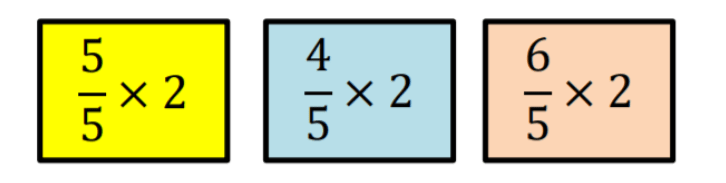Point out that we used 2 in all three multiplication problems, and multiplied it to different scaling factors, that is, 5⁄5, 4⁄5 and 6⁄5. Ask students to reflect on the following questions:

• Is the size of the product affected by the size of one of its factors?
• What will happen to the size of the product if a number is multiplied by a scaling factor equal to one?
• What will happen to the size of the product if a number is multiplied by a scaling factor greater than one?
• What will happen to the size of the product if a number is multiplied by a scaling factor less than one?

To answer these questions, go through each multiplication problem separately.

#### Multiplication Problem 1:

Write the first multiplication problem: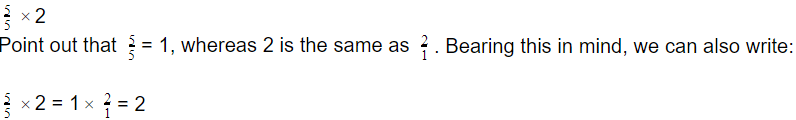Explain that by using the standard algorithm, we can conclude that when we multiply a number by a scaling factor equal to one, the size of the product does not change. It remains the same. From what we saw, the product is 2, just like the number multiplied by the scaling factor.

#### Multiplication Problem 2:

Write the second multiplication problem: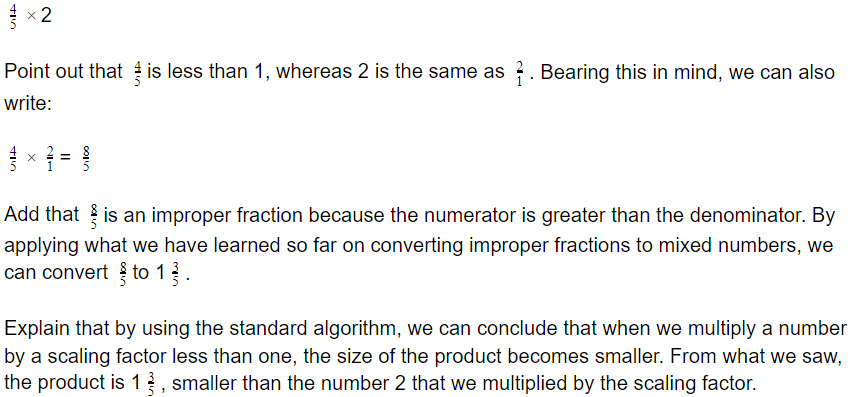#### Multiplication Problem 3:

Write the third multiplication problem: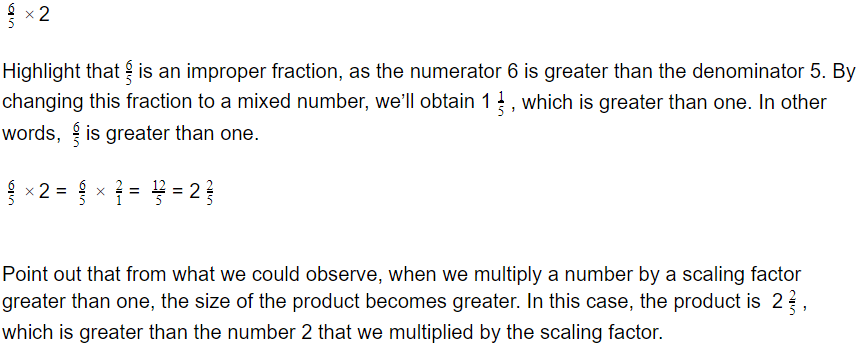If you have the technical means in your classroom, you can also complement your lesson on interpreting multiplication with multimedia materials, such as videos.

For instance, use this video by Khan Academy to provide an introduction to interpreting multiplication as scaling with fractions. The video contains worked examples on interpreting multiplication, with three multiplication problems:2⁄3 × 7⁄8, 8⁄7 × 2⁄3 and 5⁄5 × 2⁄3.

This video is also an excellent resource with guidelines on interpreting multiplication as scaling with fractions, as well as practical examples on how the numbers and fractions in different multiplication problems affect the product.

When it comes to expressing fractions as equivalent decimals, play this excellent video with simple instructions and examples. And finally, use this video that consists of a song on converting fractions to decimals to make the lesson more fun.## Activities to Practice Interpreting Multiplication

### Fraction Multiplication as Scaling Online Game

This is a simple online game developed by Khan Academy where students get to practice interpreting multiplication as scaling with fractions. To implement this activity in your class, make sure that there is a suitable device for each student.

Students play the game individually. Explain that in each exercise, students have to interpret multiplication as scaling, by comparing the size of a product to the size of one factor, given the size of the other factor, without calculation.

If they get stuck, they can also use a hint or play a video with instructions on this topic. Once students complete the exercises, provide space for discussion and reflection. Which exercises were the most challenging? Why?

### Fractions to Decimals Hearts

This is a fun game that will help students master expressing fractions to equivalent decimals, a crucial skill that students use in interpreting multiplication. To use this activity in your class, you’ll need red construction paper, markers, and some scissors.

Draw hearts on the construction paper and cut them in half. One half contains a fraction and the other half contains its equivalent decimal. For instance, if you write 1⁄5 on one half, the other half should contain 0.2.

Hand out one half to each student and provide instructions. Students have to go around the classroom and find their ‘half’, by applying their knowledge of expressing fractions to equivalent decimals.

Provide a few minutes for each student to find their ‘half’. You can also use a timer. Once you say ‘stop’, students stop moving and you check how many have managed to find their ‘half’. Which steps did they follow to convert the fractions to decimals? Did they rely on mental math?

### Scaling Fractions by Fractions Online Game

In this simple online game developed by IXL, students can practice interpreting multiplication. To implement this activity in your class, the only material needed is a suitable device for each student.

Pair students up and provide instructions for the game. Students are presented with different exercises where they have to choose the correct symbol (greater than, less than, or equal) to fill in the given expression.

Students are not supposed to calculate, but simply compare the size of a product to the size of one factor, given the size of the other factor. Students in each pair do the exercises individually. Provide some 10 – 15 minutes for students to complete the game.

After they’re done, students in each pair discuss their results with their partner and share how they approached the problems. Encourage students to discuss the steps they used in completing the exercises. By doing this, they will also have the opportunity to engage in peer tutoring.

### Puppy Chase Decimals

In this awesome online game, students can attain fluency in expressing fractions to equivalent decimals, which is often necessary when interpreting multiplication. The only material needed to use the Puppy Chase Decimals in your class are technical devices for students.

Students play the game individually, which makes it suitable for parents who are homeschooling their kids. Explain that the game consists of a doggy derby, where students have to compete against other puppies to win the doggy derby.

Players are presented with several questions where they have to convert fractions to equivalent decimals, including fractions that are mixed numbers. Students must answer correctly and as fast as possible to increase their chances of winning the derby.

In the end, encourage a discussion. What was the final score students obtained? Did students multiply the denominator of the given fraction by a number that made it 10, 100, 1 000, 10 000 (powers of ten)? Did some fractions already have a denominator of such kind?

### Interpreting Multiplication With Color Circles

This is an awesome activity that will get your students coloring and having fun while honing their ability to interpret multiplication as scaling. To use this activity in your class, you’ll need to print out this Interactive Notebook Worksheet (Members Only), and colored pencils.

Divide students into groups of 3 or 4. Hand out one copy of the worksheet to each student, as well as the colored pencils. Provide instructions for the activity. Students in each group cooperate to solve the exercises.

Explain that the exercise consists of two rows: in the first row, students have to interpret the multiplication without calculating. The second row consists of an empty circle right next to the multiplication problem. Each multiplication problem has one boxed number (a number in a box).

Students color the circle in the second row in the following ways: red – if the product is greater than the boxed number; yellow – if the product is less than the boxed number; and blue – if the product is equal to the boxed number.

Provide a few minutes for students to complete the exercises and then have the groups present their results. How did they decide which color to use for which circle? What happened to the product when the scaling factor was equal to, greater than, or less than one?

## Before You Leave…

If you enjoyed these strategies and activities for teaching interpreting multiplication, you may want to check out our lesson that’s dedicated to this topic! So if you need guidance to structure your class and teach it, sign up for our emails to receive loads of free content!

Feel free to also check out our blog – you’ll find plenty of awesome resources that you can use in your class! And if you’re ready to become a member, simply join our Math Teacher Coach community!